## 机器学习实战教程（九）：模型泛化

news/2023/12/4 21:44:28

# 模型评价与选择

## 差错分析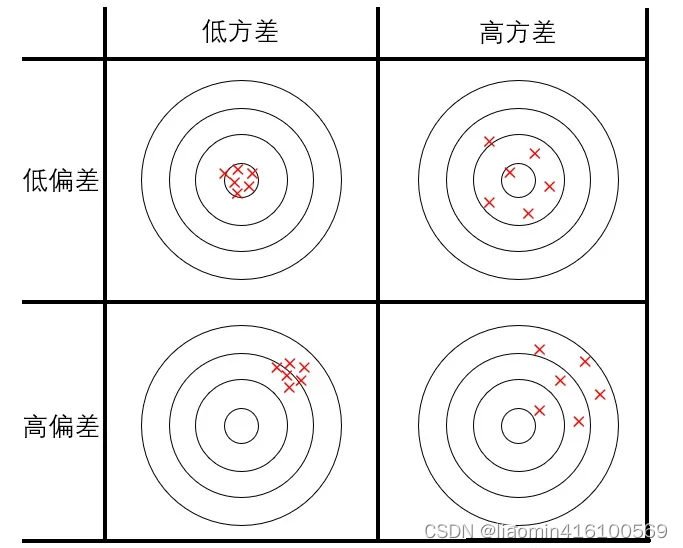• 欠拟合（underfit）：参数过少，假设函数太不自由，过于简单，连样本集都拟合不好，预测时容易偏向一侧，偏差大。
• 过拟合（overfit）：参数过多，假设函数太自由，不抗干扰，对样本集拟合得很好，但是假设函数过于畸形，预测时忽左忽右，方差大。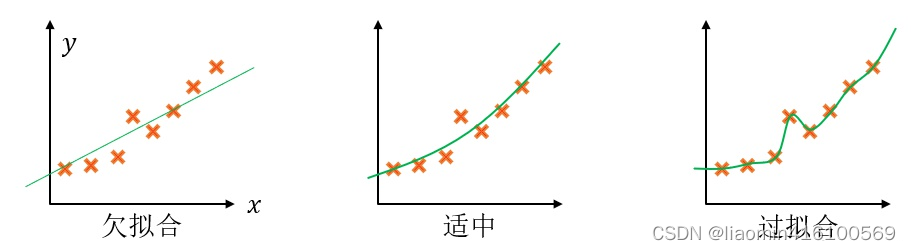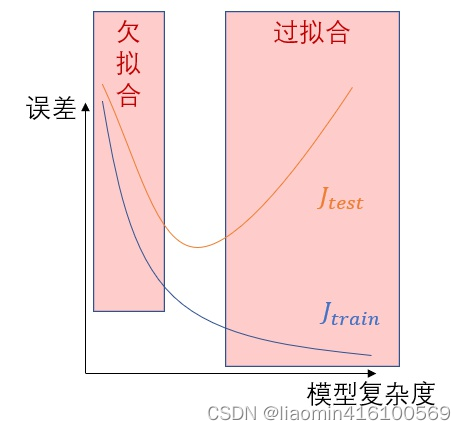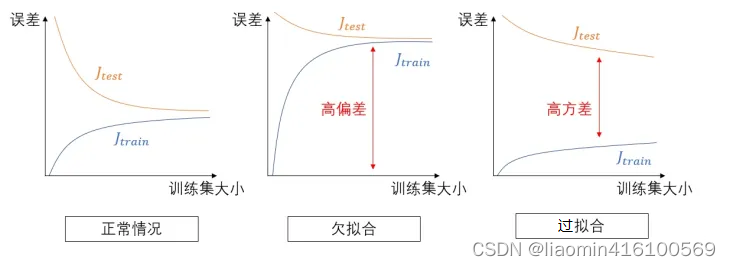• 减少该模型的参数，或者改为更简单的模型。
• 正则化。
• 增大训练集，减少噪音成分等。

## 泛化误差

θ \theta 代表超参数， J 未知 J_{未知} { \{ θ \theta } \} 代表训练出模型 θ \theta 参数后对于未知数据的误差，越小泛化能力越强， J t e s t J_{test} { \{ θ \theta } \} 代表模型对测试机的误差，越小泛化能力越强。

## 交叉验证

### 交叉验证的步骤

1. 把训练集进一步分为子训练集与交叉验证集。把测试集藏好，先不用它。（测试集是对未知数据的模拟）
2. 使用各种不同的模型在子训练集上训练，并测出各模型在交叉验证集上的 J c v J_{cv} { \{ θ \theta } \}
3. 选择 J c v J_{cv} { \{ θ \theta } \} 最小的模型，认为它最佳。把子训练集和交叉验证集合并为训练集，训练出最终的模型。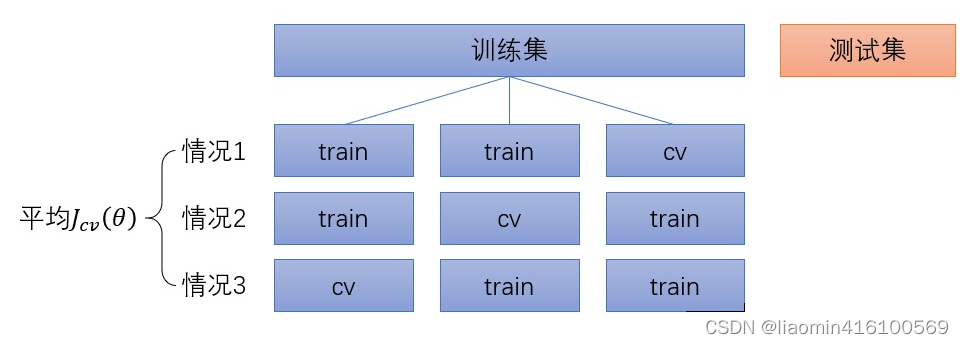K折交叉验证的优点是进一步确保交叉验证集没有特殊性，对泛化误差的估计更为准确。

### KFold拆分

from sklearn.model_selection import KFold
import numpy as np# 创建一个包含10个元素的数组作为样本数据
X = np.array([1, 2, 3, 4, 5, 6, 7, 8, 9, 10])
# 定义K值
k = 5# 创建KFold对象，并指定n_splits参数为K
kf = KFold(n_splits=k)# 遍历KFold对象中的每一组训练集和测试集
for train_index, test_index in kf.split(X):print("train_index:", train_index, "test_index:", test_index)


train_index: [2 3 4 5 6 7 8 9] test_index: [0 1]
train_index: [0 1 4 5 6 7 8 9] test_index: [2 3]
train_index: [0 1 2 3 6 7 8 9] test_index: [4 5]
train_index: [0 1 2 3 4 5 8 9] test_index: [6 7]
train_index: [0 1 2 3 4 5 6 7] test_index: [8 9]


fold的值也就决定了最后使用cv数据集验证的得分个数。

### cross_val_score实战

cross_val_score函数是Scikit-learn库中用于评估模型性能的快速方法之一。它计算基于交叉验证的模型评分，并返回每个fold的测试性能得分。与KFold不同，cross_val_score不需要显示拆分数据集。您只需提供模型和数据集即可进行评估，该函数将自动处理交叉验证过程，从而使代码更加简洁和易于理解。

#### 数据集和模型

load_digits 是 Scikit-learn 库中的一个函数，用于加载手写数字图像数据集。这个数据集包含 8x8 像素大小的 1797 张手写数字图像，每张图像都对应一个 0 到 9 的数字标签。

from sklearn.datasets import load_digitsdigits = load_digits()
import matplotlib.pyplot as pltfig, axes = plt.subplots(nrows=1, ncols=5, figsize=(10, 3))for i, ax in enumerate(axes):ax.imshow(digits.images[i], cmap='gray')ax.set_title(digits.target[i])plt.show()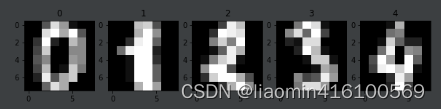• n_neighbors（即k）：指定要考虑的最近邻居的个数。默认情况下，它为5，表示预测一个新样本时将使用数据集中距离其最近的5个数据点的标签,5个中最多的那个标签就是当前数据的标签。
• p：用于计算距离的指标。默认情况下，使用Minkowski距离，p 为2，表示使用欧几里得距离。不同的 p 值对应不同的距离度量方式，例如，p=1 表示曼哈顿距离，p=3 可以使用一种更为复杂的曼哈顿距离度量方式。

#### 使用数据集和测试集获取最佳k，p

import numpy as np
from sklearn import datasets
from sklearn.model_selection import train_test_split
from sklearn.neighbors import KNeighborsClassifierdigits = datasets.load_digits()
x = digits.data
y = digits.targetx_train, x_test, y_train, y_test = train_test_split(x, y, test_size=0.4, random_state=666)best_score, best_p, best_k = 0, 0, 0
for k in range(2, 11):for  p in range(1, 6):knn_clf = KNeighborsClassifier(weights="distance", n_neighbors=k, p=p)knn_clf.fit(x_train, y_train)score = knn_clf.score(x_test, y_test)if score > best_score:best_score, best_p, best_k = score, p, kprint("Best K=", best_k)
print("Best P=", best_p)
print("Best score=", best_score)


Best K= 3
Best P= 4
Best score= 0.9860917941585535


#### 使用交叉验证获取最佳k，p

cross_val_score函数默认使用的交叉验证方法是3-Fold交叉验证，即将数据集分为3个相等的部分，其中2个部分用于训练，1个部分用于测试。在每个fold迭代中，使用测试集得到性能度量得分，然后将所有fold的结果平均并返回。

best_score, best_p, best_k = 0, 0, 0
for k in range(2, 11):for  p in range(1, 6):knn_clf = KNeighborsClassifier(weights="distance", n_neighbors=k, p=p)scores = cross_val_score(knn_clf, x_train, y_train)score = np.mean(scores)if score > best_score:best_score, best_p, best_k = score, p, kprint("Best K=", best_k)
print("Best P=", best_p)
print("Best score=", best_score)

Best K= 2
Best P= 2
Best score= 0.9823599874006478


best_knn_clf = KNeighborsClassifier(weights='distance', n_neighbors=2, p=2)
best_knn_clf.fit(x_train, y_train)
best_knn_clf.score(x_test, y_test)


## 正则化（regularization）

### 原理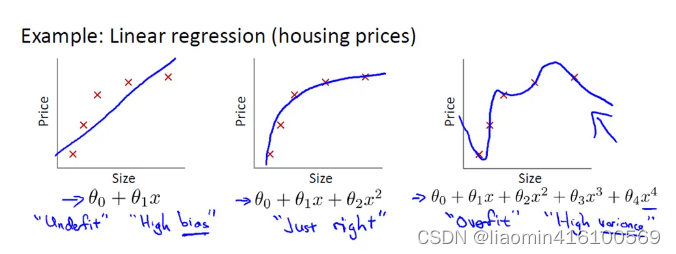θ 0 + θ 1 x + θ 2 x 2 + θ 3 x 3 + θ 4 x 4 \theta_{0}+\theta_{1}x+\theta_{2}x^2+\theta_{3}x^3+\theta_{4}x^4

( 1 2 m [ ∑ i = 1 m ( h θ ( x i ) − y i ) 2 ] ) ({1\over2m}[\sum_{i=1}^{m}{(h_\theta(x^i)-y^i)^2}])

m i n ( 1 2 m [ ∑ i = 1 m ( h θ ( x i ) − y i ) 2 ] ) min({1\over2m}[\sum_{i=1}^{m}{(h_\theta(x^i)-y^i)^2}])

1 2 m [ ∑ i = 1 m ( h θ ( x i ) − y i ) 2 ] ) + λ ∑ j = 1 n θ j 2 {1\over2m}[\sum_{i=1}^{m}{(h_\theta(x^i)-y^i)^2}])+\lambda\sum_{j=1}^{n}\theta_{j}^2

shrinkage方法介绍：指训练求解参数过程中考虑到系数的大小，通过设置惩罚系数，使得影响较小的特征的系数衰减到0，只保留重要特征的从而减少模型复杂度进而达到规避过拟合的目的。常用的shinkage的方法有Lasso（L1正则化）和岭回归（L2正则化）等。
Lasso（L1正则化）公式：
1 2 m [ ∑ i = 1 m ( h θ ( x i ) − y i ) 2 ] + λ ∑ j = 1 n ∣ θ j ∣ {1\over2m}[\sum_{i=1}^{m}{(h_\theta(x^i)-y^i)^2}]+\lambda\sum_{j=1}^{n}|\theta_{j}|

1. 一方面因为模型可能考虑到很多没必要的特征，这些特征对于模型来说就是噪声，shrinkage可以通过消除噪声从而减少模型复杂度；
2. 另一方面模型特征存在多重共线性（变量之间相互关联）的话可能导致模型多解，而多解模型的一个解往往不能反映模型的真实情况，shrinkage可以消除关联的特征提高模型稳定性。

### 对应图形

J = J 0 + λ ∑ w w 2 J=J_{0}+\lambda\sum_ww^2
J0表示原始的损失函数，咱们假设正则化项为：

L = λ ( w 1 2 + w 2 2 ) L=\lambda(w_{1}^2+w_{2}^2)

( x − a ) 2 + ( y − b ) 2 = r 2 (x-a)^2+(y-b)^2=r^2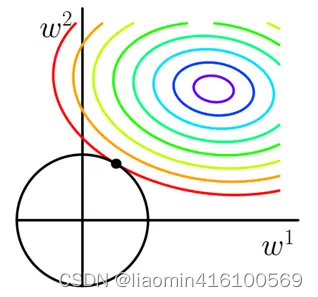L表示为图中的黑色圆形，随着梯度下降法的不断逼近，与圆第一次产生交点，而这个交点很难出现在坐标轴上。

### 岭回归（Ridege Regression）

import numpy as np
import matplotlib.pyplot as pltnp.random.seed(42)
x = np.random.uniform(-3.0, 3.0, size=100)
X = x.reshape(-1, 1)
y = 0.5 * x + 3 + np.random.normal(0, 1, size=100)plt.scatter(x, y)
plt.show()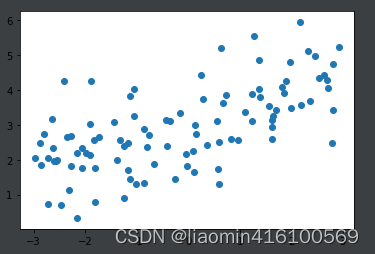from sklearn.linear_model import LinearRegression
from sklearn.pipeline import Pipeline
from sklearn.preprocessing import PolynomialFeatures
from sklearn.preprocessing import StandardScaler
from sklearn.model_selection import train_test_split
from sklearn.metrics import mean_squared_errordef PolynomiaRegression(degree):return Pipeline([('poly', PolynomialFeatures(degree=degree)),('std_scale', StandardScaler()),('lin_reg', LinearRegression()),])np.random.seed(666)
x_train, x_test, y_train, y_test = train_test_split(X, y)poly_reg = PolynomiaRegression(degree=20)
poly_reg.fit(x_train, y_train)y_poly_predict = poly_reg.predict(x_test)
print(mean_squared_error(y_test, y_poly_predict))
# 167.9401085999025
import matplotlib.pyplot as plt
x_plot = np.linspace(-3, 3, 100).reshape(100, 1)
y_plot = poly_reg.predict(x_plot)plt.scatter(x, y)
plt.plot(x_plot[:,0], y_plot, color='r')
plt.axis([-3, 3, 0, 6])
plt.show()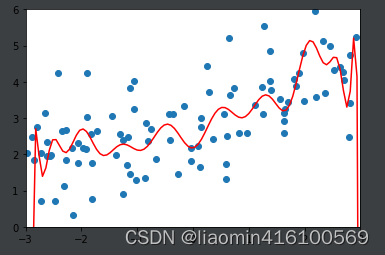def plot_model(model):x_plot = np.linspace(-3, 3, 100).reshape(100, 1)y_plot = model.predict(x_plot)plt.scatter(x, y)plt.plot(x_plot[:,0], y_plot, color='r')plt.axis([-3, 3, 0, 6])plt.show()


from sklearn.linear_model import Ridge
def RidgeRegression(degree, alpha):return Pipeline([('poly', PolynomialFeatures(degree=degree)),('std_scale', StandardScaler()),('lin_reg', Ridge(alpha=alpha)),])ridege1_reg = RidgeRegression(20, alpha=0.0001)
ridege1_reg.fit(x_train, y_train)y1_predict = ridege1_reg.predict(x_test)
print(mean_squared_error(y_test, y1_predict))
# 跟之前的136.相比小了很多
plot_model(ridege1_reg)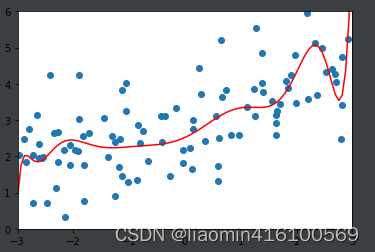ridege2_reg = RidgeRegression(20, alpha=1)
ridege2_reg.fit(x_train, y_train)y2_predict = ridege2_reg.predict(x_test)
print(mean_squared_error(y_test, y2_predict))
plot_model(ridege2_reg)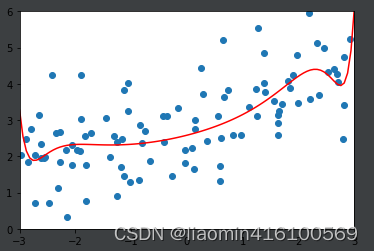ridege2_reg = RidgeRegression(20, alpha=100)
ridege2_reg.fit(x_train, y_train)y2_predict = ridege2_reg.predict(x_test)
print(mean_squared_error(y_test, y2_predict))
# 1.3196456113086197
plot_model(ridege2_reg)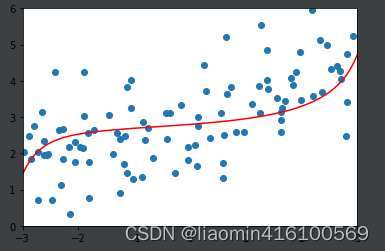ridege2_reg = RidgeRegression(20, alpha=1000000)
ridege2_reg.fit(x_train, y_train)y2_predict = ridege2_reg.predict(x_test)
print(mean_squared_error(y_test, y2_predict))
# 1.8404103153255003
plot_model(ridege2_reg)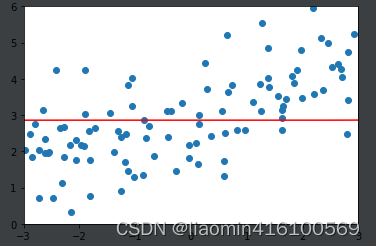### LASSO Regularization

#%%import numpy as np
import matplotlib.pyplot as plt
from skimage.metrics import mean_squared_error
from sklearn.model_selection import train_test_split
from sklearn.pipeline import Pipeline
from sklearn.preprocessing import PolynomialFeatures, StandardScaler
np.random.seed(42)
x = np.random.uniform(-3.0, 3.0, size=100)
X = x.reshape(-1, 1)
y = 0.5 * x + 3 + np.random.normal(0, 1, size=100)
np.random.seed(666)
x_train, x_test, y_train, y_test = train_test_split(X, y)plt.scatter(x, y)
plt.show()#%%from sklearn.linear_model import Lasso
def plot_model(model):x_plot = np.linspace(-3, 3, 100).reshape(100, 1)y_plot = model.predict(x_plot)plt.scatter(x, y)plt.plot(x_plot[:,0], y_plot, color='r')plt.axis([-3, 3, 0, 6])plt.show()
def LassoRegression(degree, alpha):return Pipeline([('poly', PolynomialFeatures(degree=degree)),('std_scale', StandardScaler()),('lin_reg', Lasso(alpha=alpha)),])
def TestRegression(degree, alpha):lasso1_reg = LassoRegression(degree, alpha) #这里相比Ridge的alpha小了很多，这是因为在Ridge中是平方项lasso1_reg.fit(x_train, y_train)y1_predict = lasso1_reg.predict(x_test)print(mean_squared_error(y_test, y1_predict))# 1.149608084325997plot_model(lasso1_reg)


TestRegression(20,0.01)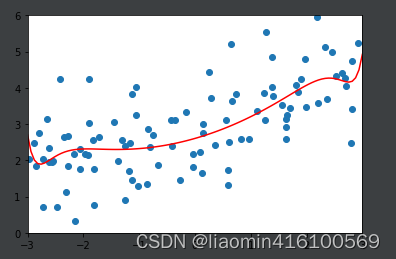TestRegression(20,0.1)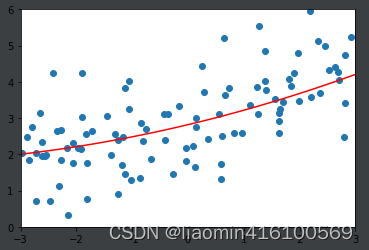TestRegression(20,1)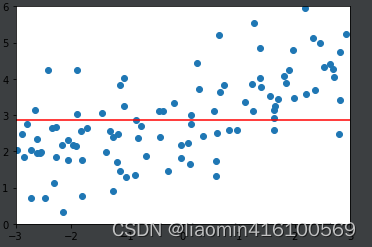### 解释Ridge和LASSO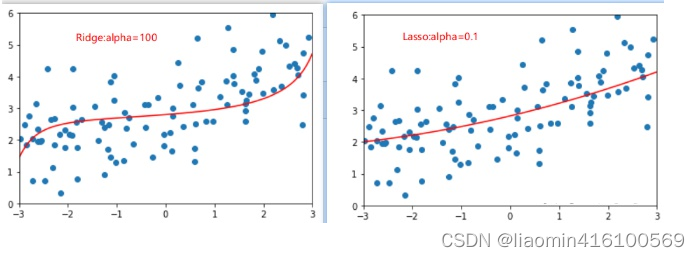### rk3568 适配摄像头 (mipi 单摄)

rk3568 适配摄像头 (mipi 单摄) MIPI CSI&#xff08;Mobile Industry Processor Interface Camera Serial Interface&#xff09;是一种用于移动设备的高速串行接口标准&#xff0c;用于连接图像传感器和图像处理器。MIPI CSI接口使用差分信号传输技术&#xff0c;将数据分为…

### 【链表】力扣203题：移除链表元素

【链表】力扣203题&#xff1a;移除链表元素 力扣203题&#xff1a;移除链表元素 建议在看题目之前先了解数组的具体知识点&#xff0c;可以看这里&#xff1a; 算法基础&#xff08;三&#xff09;&#xff1a;链表知识点及题型讲解。 其它题目&#xff1a; 【链表】力扣206题…

### vue---mixin混入

mixins是一种分发 Vue 组件中可复用功能的非常灵活的方式。混合对象可以包含任意组件选项。当组件使用混合对象时&#xff0c;所有混合对象的选项将被混入该组件本身的选项。 一个混入对象可以包含任意组件选项&#xff08;如data、methods、created、mounted等等&#xff09;。…

### Stable Diffusion XL：更快，更强

Stable Diffusion XL&#xff1a;更快&#xff0c;更强 今天&#xff0c;Stability AI 的创始人兼首席执行官 Emad Mostaque 发推宣布&#xff0c;Stable Diffusion XL 进入公测阶段。 核心信息总结起来有2点&#xff1a; “XL”不是新模型的官方名称&#xff0c;Stability …

### 【cuda】Nsight System 下载，安装与使用

Nsight System 下载 nsys 是 NVIDIA Nsight Systems 的命令行工具&#xff0c;可以用于分析 CUDA 应用程序的性能和行为。以下是在 Linux 上安装 nsys 的步骤&#xff1a; 下载 NVIDIA Nsight Systems 安装程序。您可以在 NVIDIA 的官方网站上下载适用于您的系统的安装程序。…

### 手推A Unified Solution to Constrained Bidding in Online Display Advertising论文

A Unified Solution to Constrained Bidding in Online Display Advertising&#xff1a;一种对在线展示广告约束出价问题的通用解决方案 未开放但是可以搜到 NeuralAuction: 电商广告中的端到端机制优化方法 https://arxiv.org/abs/2106.03593 一种使用真负样本的在线延迟反…

### 【LeetCode】650. 只有两个键的键盘

650. 只有两个键的键盘&#xff08;中等&#xff09; 思路 不同于以往通过加减实现的动态规划&#xff0c;这里需要乘除法计算位置。因为粘贴操作是倍数增加&#xff0c;使一个一维数组 dp&#xff0c;其中位置 i 表示延展到长度 i 的最少操作次数。对于每个位置 j &#xff0c…

### WMS是什么？

WMS&#xff08;Warehouse Management System&#xff09;中文译作仓库管理系统&#xff0c;是一种专用于物流仓储管理的IT系统。它主要应用于企业物流中心、配送中心、供应商物料储备中心、电子商务配送中心等仓库管理过程中。 WMS系统可以帮助企业管理和控制其物流仓储流程。…